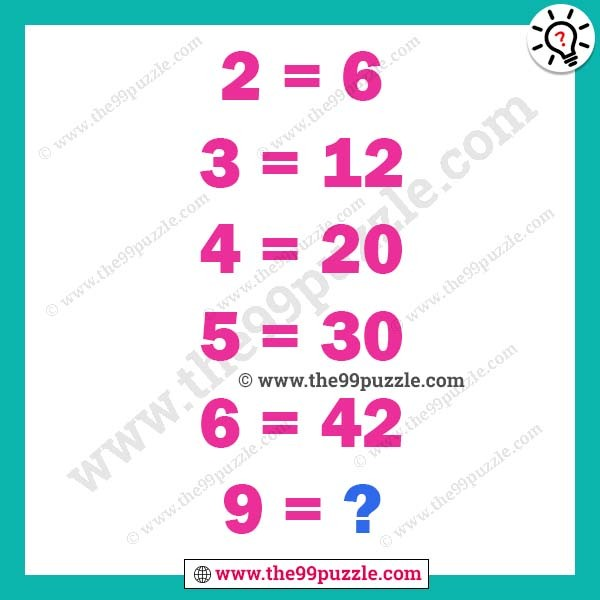Brain teaser math riddle for adult with answer. Here this puzzle picture gives some equations. If you are a genius in math puzzles you can easily find out the math logic. These math puzzles question improves the logical thinking. Think logically and mathematically to find out the last equations.

2 = 6

3 = 12

4 = 20

5 = 30

6 = 42

9 = ?2=(2×3)=6

3=(3×4)=12

4=(4×5)=20

5=(5×6)=30

6 =(6×7)=42

9 =(9×10)=90